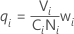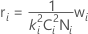# Calculations for the mean pool and the variance pool for Allocate Gap Pools

## Allocate the mean pool

whereIf you do not specify mean shift factors, then the mean pool = 0.

## Allocate the variance pool

Calculate adjusted standard deviations as follows:

whereIf you do not specify variation expansion factors and you do not specify both gap specification limits, then the variance pool = 0.

## Notation

TermDescription
CiDiametrical correction of the ith element
DiDrift factor for the ith element
NiComplexity of the ith element
SiShift factor for the ith element
σiStandard deviation of the ith element